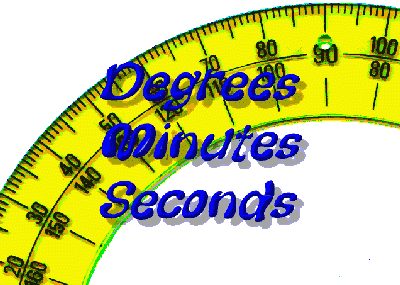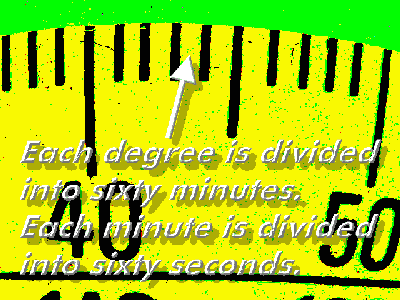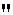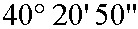Degrees, Minutes, Seconds

Calculators at the bottom of this page.There are several ways to measure the size of an angle. One way is to use units of degrees. (Radian measure is another way.) In a complete circle there are three hundred and sixty degrees. An angle could have a measurement of 35.75 degrees. That is, the size of the angle in this case would be thirty-five full degrees plus seventy-five hundredths, or three fourths, of an additional degree. Notice that here we are expressing the measurement as a decimal number. Using decimal numbers like this one can express angles to any precision - to hundredths of a degree, to thousandths of a degree, and so on. There is another way to state the size of an angle, one that subdivides a degree using a system different than the decimal number example given above. The degree is divided into sixty parts called minutes. These minutes are further divided into sixty parts called seconds. The words minute and second used in this context have no immediate connection to how those words are usually used as amounts of time.
 In a full circle there are 360 degrees. Each degree is split up into 60 parts, each part being 1/60 of a degree.  These parts are called minutes. Each minute is split up into 60 parts, each part being 1/60 of a minute. These parts are called seconds.The size of an angle could be stated this way: 40 degrees, 20 minutes, 50 seconds.

There are symbols that are used when stating angles using degrees, minutes, and seconds. Those symbols are show in the following table.

 Symbol for degree:Symbol for minute:Symbol for second:So, the angle of 40 degrees, 20 minutes, 50 seconds is usually written this way:How could you state the above as an angle using common decimal notation? The angle would be this many degrees, (* means times.):

 40 + (20 * 1/60) + (50 * 1/60 * 1/60)

That is, we have 40 full degrees, 20 minutes - each 1/60 of a degree, and 50 seconds - each 1/60 of 1/60 of a degree.

Work that out and you will get a decimal number of degrees.  It's 40.34722...

Going the other way is a bit more difficult. Suppose we start with 40.3472 degrees. Can we express that in units of degrees, minutes, and seconds?

Well, first of all there are definitely 40 degrees full degrees. That leaves 0.3472 degrees.

So, how many minutes is 0.3472 degrees? Well, how many times can 1/60 go into 0.3472? Here's the same question: What is 60 times 0.3472?  It's 20.832. So, there are 20 complete minutes with 0.832 of a minute remaining.

How many seconds are in the last 0.832 minutes. Well, how many times can 1/60 go into 0.832, or what is 60 times 0.832? It's 49.92, or almost 50 seconds.

So, we've figured that 40.3472 degrees is almost exactly equal to 40 degrees, 20 minutes, 50 seconds.

(The only reason we fell a bit short of 50 seconds is that we really used a slightly smaller angle in this second half of the calculation explanation.  In the original angle, 40.34722... degrees, the decimal repeats the last digit of 2 infinitely, so, the original angle is a bit bigger than 40.3472.)

 Degrees: Minutes: Seconds:

Here's a calculator that will take you from angle measurement using degrees, minutes, and seconds to angle measurement expressed as a decimal number.

Make up an angle measurement using degrees, minutes, and seconds. Then, using the above procedures, calculate what that angle measurement would be if it were expressed as a decimal number. Finally, enter your original angle in degrees, minutes, and seconds into this calculator, click the 'Calculate' button, and see if your conversion mathematics was correct.

 Decimal number of degrees:

Here's another calculator that you can use to check your work going from an angle measurement using a decimal number of degrees to the same angle measurement expressed in terms of degrees, minutes, and seconds. The final result is rounded to the nearest second.